SAT II Math I : Probability

Example Questions

2 Next →

Example Question #152 : Data Analysis And Statistics

If you draw an ace from and deck then place it back in the deck, what is the probability of drawing another ace?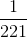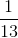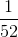Explanation:

Since the first card was placed back into the deck, it will have no effect on the second draw.

Since there areaces in a deck ofcards, the probability would be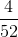or.

Example Question #153 : Data Analysis And Statistics

A fair 6 sided die is rolled twice. What is the probability of observing a 6 both times?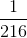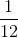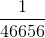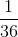Explanation:

The probability of rolling a 6 once isbecause 6 is one of 6 different possible outcomes.

The probability of two independent events happening can be multiplied together. The probability of getting 2 6's is: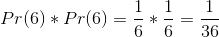Example Question #154 : Data Analysis And Statistics

If an integeris randomly selected such that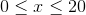, what is the probability thatis a prime number?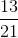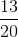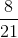Explanation:

Probability is defined as specified outcomes divided by total possible outcomes.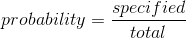Recall that a prime numbered is defined as a number that is divisible only by one and itself, andis not prime. There areprime numbers in the range of possiblevalues: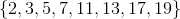Notice that the range of values,includes zero , so there aretotal numbers to select from.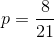2 Next →